nextnano.com  GUI: nextnanomat  Tool: nextnano++  Tool: nextnano³  Tool: nextnano.NEGF  Download | Search | Copyright | News | Publications  * password protected nextnano³ softwareBIO-1D GaN/AlGaN electrolyte

# nextnano3 - Tutorial

## ISFET (Ion-Selective Field Effect Transistor): Electrolyte Gate AlGaN/GaN Field Effect Transistor as pH Sensor

Author: Stefan Birner

If you want to obtain the input file that is used within this tutorial, please contact stefan.birner@nextnano.de.
```-> 1DGaN_electrolyte_sensor.in  ```

This tutorial is based on the following paper
Theoretical study of electrolyte gate AlGaN/GaN field effect transistors
M. Bayer, C. Uhl, P. Vogl
Journal of Applied Physics 97 (3), 033703, (2005)
as well as on the diploma theses of both Christian Uhl and Michael Bayer.
Note that this tutorial only briefly sketches the underlying physics. So please check these references for more details.

Acknowledgement: The author - Stefan Birner - would like to thank Christian Uhl and Michael Bayer for helping to include the electrolyte features into nextnano³.

## Electrolyte Gate AlGaN/GaN Field Effect Transistor as pH Sensor

Here, we predict the sensitivity of electrolyte gate AlGaN/GaN field effect transistors (FET) to pH values of the electrolyte solution that covers the semiconductor structure. Particularly, we need to take into account the piezo- and pyroelectric polarization fields according to the pseudomorphic growth of the nitride heterostructure on a sapphire substrate (However, here we assume that the heterostructure is strained with respect to GaN).
The charge density due to chemical reactions at the oxidic semiconductor-electrolyte interface is described within the site-binding model (`\$interface-states`).
We calculate the spatial charge and potential distribution both in the semiconductor and the electrolyte (Poisson-Boltzmann equation) self-consistently.

The AlGaN/GaN FET that is exposed to an electrolyte solution has the following schematic layout: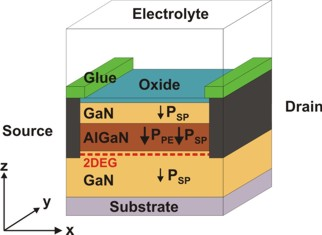Fig. 1: AlGaN/GaN FET with electrolyte gate.
The polarization charges are included for the case of Ga-face polarity.
The polarization fields lead to the formation of a 2DEG.

We simulate the structure along the z direction and neglect source and drain so that the structure is effectively one-dimensional, i.e. laterally homogeneous.
The nitride heterostructure is assumed to be grown along the hexagonal  direction and is of Ga-face polarity. The AlGaN layer is strained with respect to GaN but the remaining layers are unstrained.
The piezo- and pyroelectric polarization of wurtzite GaN and Al0.28Ga0.72N result in huge polarization fields within the structure. The divergence of the total polarization across the interface between adjacent layers causes a fixed interfacial sheet charge density.
The following figure shows the influence of the interface charge densities: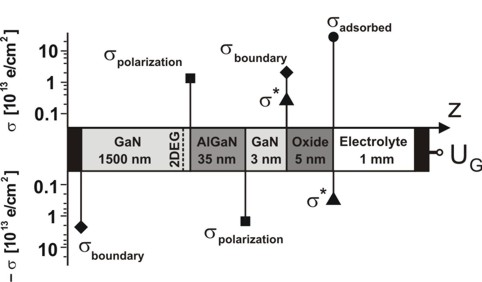Fig. 2: Schematic layout of the calculated AlGaN/GaN heterostructure including the interface charge densities. The magnitudes are indicated by the filled symbols.

The file``` densities/interface_densitiesD.txt ```gives us information about the relevant interface charge densities:

• Interface 1 (1 nm): sigmaboundary = - 2.2 * 1013 e / cm²
as defined in the input file:
``` \$interface-states   state-number         = 1            ! between Metal / GaN at 1 nm   state-type           = fixed-charge ! -sigma_boundary   interface-density    = -2.2d13      ! -2.2 x 10^13 [|e|/cm^2]```
• Interface 2 (1500 nm): sigmapolarization (Interface 2) = sigmapiezo + sigmapyro = 1.38 * 1013 e / cm²
```                                              -> ```sigmapiezo = 6.61 * 1012 e / cm²
```                                              -> ```sigmapyro = 7.14 * 1012 e / cm²
• Interface 3 (1535 nm): sigmapolarization (Interface 3)  = - sigmapolarization  (Interface 2)
• Interface 4 (1538 nm): sigmaboundary as for Interface 1 (but with different sign) plus an additional charge sigma* = 1.0 * 1013 e / cm² as defined in the input file:
``` \$interface-states   state-number         = 3            ! between GaN / Oxide at 1538 nm   state-type           = fixed-charge ! sigma*   interface-density    = 1.0d13       ! 1 x 10^13 [|e|/cm^2]```
• Interface 5 (1543 nm): sigma* as for Interface 4 (but with different sign) plus an additional charge sigmaadsorbed that results from the site-binding model that describes chemical reactions at the oxidic semiconductor-electrolyte interface. More details: ``` \$interface-states```
``` \$interface-states   state-number          = 5           ! between Oxide / Electrolyte at 1543 nm   state-type            = electrolyte ! sigma_adsorbed   interface-density     = 9.0d14      ! [cm^-2] - ```total density of surface sites, i.e. surface hydroxyl groups```   adsorption-constant   = 1.0d-8      ! K1 = adsorption   constant   dissociation-constant = 1.0d-6      ! K2 = dissociation constant  \$electrolyte   ...   pH-value              = 5.3d0       ! pH = -lg(concentration) = 5.3 -> ```concentration in``` [M]=[mol/l] ``````   ```(The point of zero charge for GaO is at pH = 6.8.)

For the origin of sigmaboundary and sigma* please refer to the references that are given above.

The GaN region (1 nm - 1500 nm) is homogeneously n-type doped with a concentration of 1 * 1016 cm-3.

The electrolyte region (1543 nm - 2999 nm) contains the following ions:
```  !---------------------------------------------------------------------------!  ! The electrolyte (NaCl, Hepes) contains four types of ions:  !   1) 100 mM singly charged cations (Na+)  !   2) 100 mM singly charged anions  (Cl-)  !   3)  10 mM doubly charged cations (Hepes2+ solution)  !   4)  20 mM singly charged anions  (Hepes-  solution)  !---------------------------------------------------------------------------!  \$electrolyte-ion-content   ion-number        = 1              ! ```100 mM singly charged cations```   ion-valency       = 1d0            ! ```charge of the ion:``` Na+   ion-concentration = 0.100d0        ! ```Input in units of:``` [M] = [mol/l] = 1d-3 [mol/cm³]   ion-region        = 1543d0  2999d0 ! ```refers to region where the electrolyte has to be applied to```   ion-number        = 2              ! ```100 mM singly charged anions```   ion-valency       = -1d0           ! ``` charge of the ion:``` Cl-   ion-concentration = 0.100d0        ! ```Input in units of:``` [M] = [mol/l] = 1d-3 [mol/cm³]   ion-region        = 1543d0  2999d0 ! ```refers to region where the electrolyte has to be applied to```   ion-number        = 3              ! ```10 mM doubly charged cations```   ion-valency       = 2d0            ! ``` charge of the ion:``` Hepes2+   ion-concentration = 0.010d0        ! ```Input in units of:``` [M] = [mol/l] = 1d-3 [mol/cm³]   ion-region        = 1543d0  2999d0 ! ```refers to region where the electrolyte has to be applied to```   ion-number        = 4              ! ```20 mM singly charged anions```   ion-valency       = -1d0           ! ``` charge of the ion:``` Hepes-   ion-concentration = 0.020d0        ! ```Input in units of:``` [M] = [mol/l] = 1d-3 [mol/cm³]   ion-region        = 1543d0  2999d0 ! ```refers to region where the electrolyte has to be applied to

In addition to these four types of ions, the pH value (as specified in` \$interface-states`) automatically determines inside the code four further types of ions, namely the concentration of H3O+, OH`-` and the corresponding anions`-` (conjugate base: [anion`-`] = 10-pH - 10-pOH = 10-5.3 - 10-8.7 = 5.01 x 10-6) and cations+ (conjugate acid; zero in this tutorial because pH = 5.3 < 7). For details, confer` \$electrolyte-ion-content`.

We have to solve the nonlinear Poisson equation over the whole device, i.e. including the Poisson-Boltzmann equation that governs the charge density in the electrolyte region.
As for the boundary conditions we assume at the right boundary (Electrolyte/Metal) a Dirichlet boundary condition where the electrostatic potential phi is equal to UG where UG is the gate voltage determined by an electrode in the electrolyte solution and which is constant throughout the entire electrolyte region. In this example the applied gate voltage is UG = 0 V. Note that the reference potential UG enters the Poisson-Boltzmann equation and also the equation for the site-binding model at the oxide/electrolyte interface. So the Dirichlet boundary condition is phi = 0 V. This corresponds to the fact that at the right part of the electrolyte,  i.e. at 'infinity' (at 2999 nm) the ion concentration is the 'equilibrium' (default) concentration as defined in``` ````\$electrolyte-ion-content`.
At the left boundary (Metal/GaN) we use a generalized Neumann boundary condition with a potential gradient that corresponds to the polarization charge -sigmaboundary = - 2.2 * 1013 e / cm². Thus the electric field E is given by
E = d phi / d z = -sigmaboundary / (epsilon0 * epsilonr) * (-1) = - 3.977 * 108 V/m.
epsilonr = `vacuum-permittivity `(see` \$physical-constants`)
epsilon0(GaN along  axis) = 10.01
Note that the generalized Neumann boundary condition is automatically taken into account as sigmaboundary is specified at the left contact. Thus it is not necessary to specify the electric field of``` -3.977d8 ```V/m.

```  \$poisson-boundary-conditions    poisson-cluster-number  = 1    region-cluster-number   = 1    boundary-condition-type = Neumann  ! Not necessary:  ! electric-field          = -3.977d8 ! -3.977 * 108 [V/m]```, corresponds to` `sigmaboundary``` = - 2.2 * 1013 [e/cm2] ```at the left boundary```  ! Specify this instead:    electric-field          = 0d0      ! 0 [V/m]    poisson-cluster-number  = 2    region-cluster-number   = 7    boundary-condition-type = Dirichlet    potential               = 0d0      ! phi = 0 [V] <=> UG = 0 [V]  ```

## Oxide/electrolyte interface potential as a function of pH value

The GaN heterostructure acts as a sensor via the semiconductor-electrolyte interface potential that reflects sigmaadsorbed, the pH value and the spatial dependence of the electrostatic potential in the solution as described by the Poisson-Boltzmann theory.

Choosing` flow-scheme = 30`, several calculations are performed while sweeping over the pH value from 0 to 12.
```    pH-value              = ```(value is overwritten internally in the program)

The file` InterfacePotential_vs_pH1D.dat `gives us the information about the electrostatic potential at the oxide/electrolyte interface for different pH values.

We performed these calculations three times where we varied the adsorption and dissociation constants.

• ```adsorption-constant   = 1.0d-8  ! K1 = adsorption   constant  (best fit to experiment) dissociation-constant = 1.0d-6  ! K2 = dissociation constant  (best fit to experiment)```
• ```adsorption-constant   = 1.0d-10 ! K1 = adsorption   constant dissociation-constant = 1.0d-10 ! K2 = dissociation constant```
• ```adsorption-constant   = 1.0d-10 ! K1 = adsorption   constant dissociation-constant = 1.0d6   ! K2 = dissociation constant```

The total density of surface sites, i.e. surface hydroxyl groups, was taken to be the same in all three cases:```     interface-density     = 9.0d14  ! [cm^-2]```

The surface potential is defined as the difference of the electrostatic potential at the oxide/electrolyte interface and the reference potential UG (Here: UG = 0 V).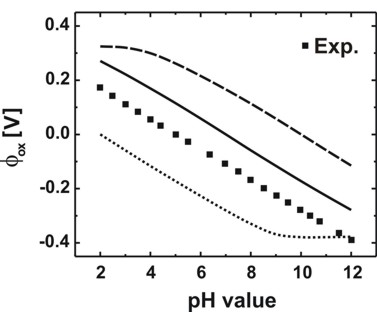Fig. 3: Calculated oxide/electrolyte interface potential as a function of the pH value.
The solid line shows the result for K1 = 10-8, K2 = 10-6.
Also included are the cases for K1 = 10-10, K2 = 10-10 (dashed line), K1 = 10-10, K2 = 106 (dotted line)
and the experimental data (G. Steinhoff et al., APL 83, 177 (2003).

Note that in Fig. 3 only the slope (d phi / d pH) is relevant but not the absolute values of the potential. The experiment gives 56.0 +/- 0.5 mV/pH. Our best fit parameters (solid line) yield 55.9 mV/pH and reproduce the constant slope over the entire pH range.

## Oxide/electrolyte interface charge density sigmaadsorbed as a function of pH value

From the file` InterfacePotentialDensity_vs_pH1D.dat `we also obtain information about the oxide/electrolyte interface sheet charge density sigmaadsorbed (as a function of pH value) that is determined by the amphoteric reactions at the oxide surface.

We plot in Fig. 4 for the following constants the oxide/electrolyte interface charge density sigmaadsorbed:
```  adsorption-constant   = 1.0d-8  ! K1 = adsorption   constant  (best fit to experiment)   dissociation-constant = 1.0d-6  ! K2 = dissociation constant  (best fit to experiment)```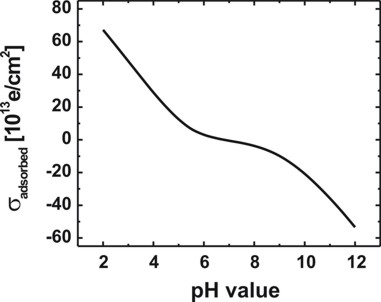Fig. 4: Calculated variation of the oxide/electrolyte interface charge density sigmaadsorbed of the amphoteric oxide surface with the pH value of the electrolyte solution.
Note that there is a range of pH values where the net surface charge density is close to zero.
The calculated point of zero charge for the GaO surface is reached for pH = 6.8.

## Electrostatic potential for different electrolyte gate voltages UG

Now we want to plot the electrostatic potential for different values of UG, i.e. for applying a gate voltage to the electrolyte. Note that UG is both the Dirichlet boundary condition for the electrostatic potential at the right contact as well as the reference potential that enters into the Poisson-Boltzmann equation (i.e. into the exponential term of the ion charge density). UG is constant throughout the entire electrolyte region.

UG =   0.5 V:
```   poisson-cluster-number  = 2    region-cluster-number   = 7    boundary-condition-type = Dirichlet    potential               =  0.5d0    ! phi =  0.5 [V] <=> UG =  0.5 [V]```

UG = - 0.5 V:
```   poisson-cluster-number  = 2    region-cluster-number   = 7    boundary-condition-type = Dirichlet    potential               = -0.5d0    ! phi = -0.5 [V] <=> UG = -0.5 [V]```

The pH value is set to 5.3.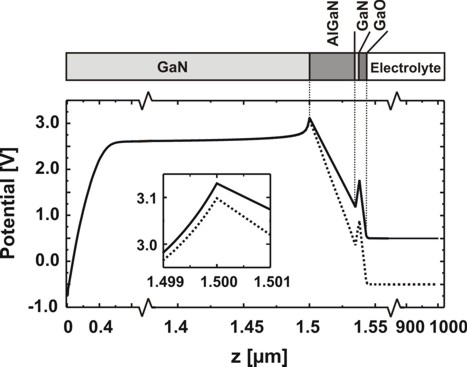Fig. 5: Spatial electrostatic potential distribution for pH = 5.3 in the electrolyte.
Depicted are the cases UG = 0.5 V (solid line) and UG = - 0.5 V (dotted line).
The inset illustrates the effect of an applied voltage on the potential near the position of the 2DEG.

• Please help us to improve our tutorial. Send comments to``` support [at] nextnano.com```.